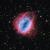The second question in Sadlers 3C Specialist textbook requires the addition and multiplication of 3 dimensional vectors.

When showing the addition of vectors, we place them end to end. The resulting vector (from the start of the first vector to the end of the last can then be determined.

The initial process outlined for showing a vector required that it always start from the origin. When adding vectors, the second vector needs to start from the end of the first. Therefore, rather than using the command HOME we can reverse the vector directions

The second issue we face is the need for 2 vectors, hence we need to define 2 vector – VECTORA and VECTORB, in the second, MAKE “A POS becomes MAKE “B POS and MAKE “A becomes MAKE “B

If we now use the command

We can see our 2 vectors placed end to end.

• To determine the resultant vector we use
• If we need to show the resultant vector,
• Note – more vectors can be added as needed.

Example